Home > CCA2 > Chapter 12 > Lesson 12.2.2 > Problem12-117

12-117.
1. Simplify each expression. Homework Help ✎

1.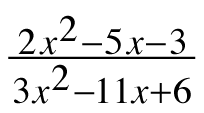2.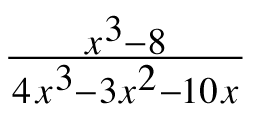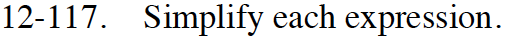$\frac{2x+1}{3x-2}$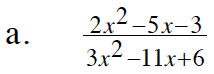Use a generic rectangle and diamond to factor the numerator
and denominator. Then simplify using Giant Ones.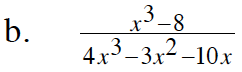The expression in the numerator is a difference of cubes.
See the Math Notes box in Lesson 8.3.3 for the formula.

When factoring the denominator,start by factoring out the common factor, x.